Lines parallel x or y axis

Chapter 4 Class 9 Linear Equations in Two Variables
Concept wise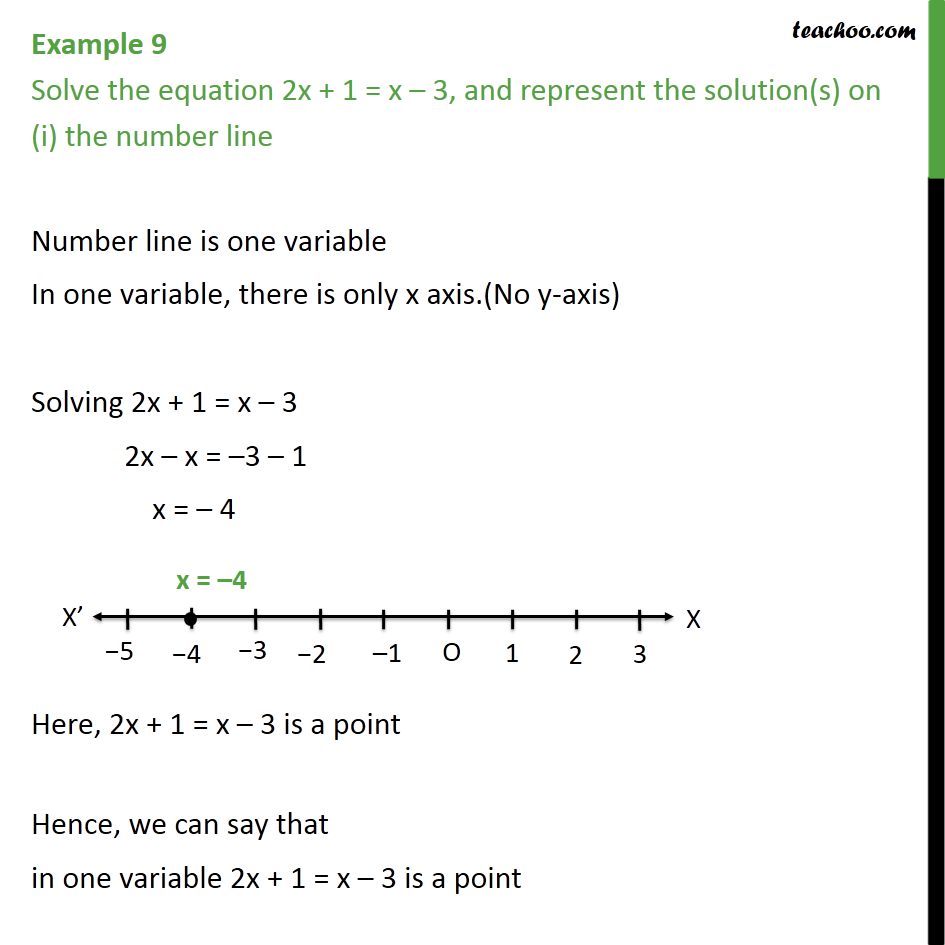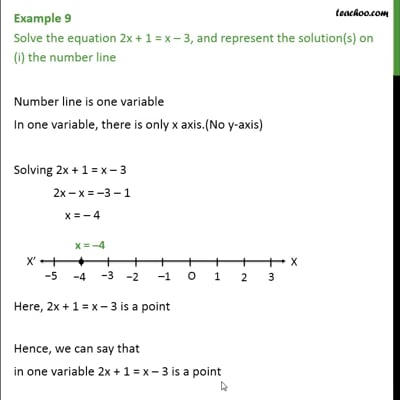This video is only available for Teachoo black users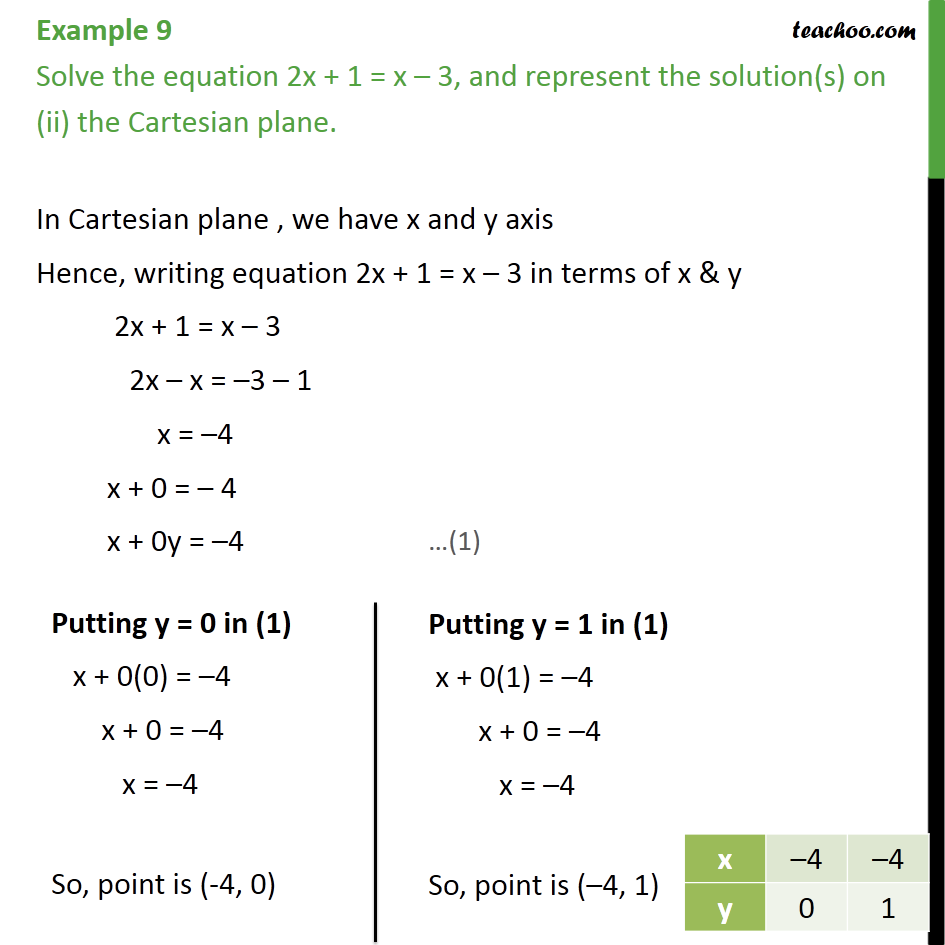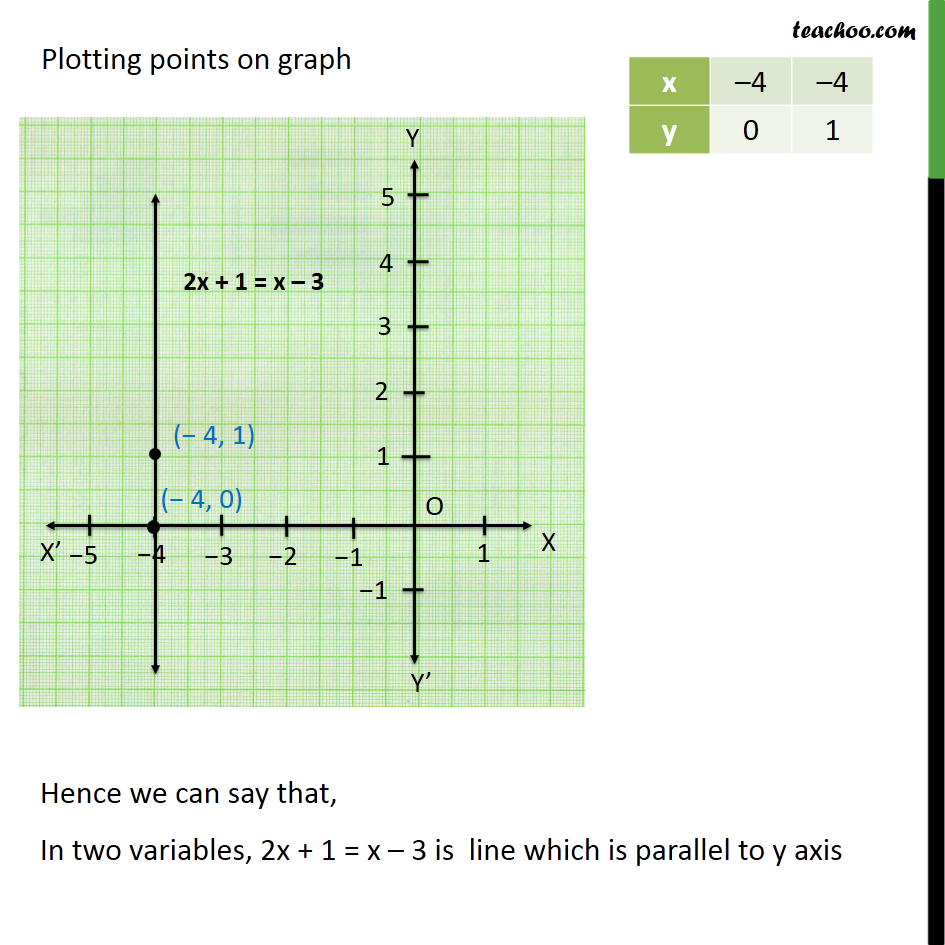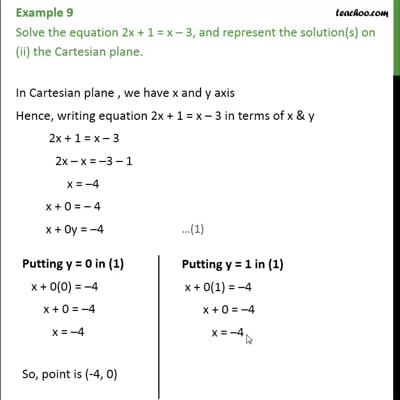This video is only available for Teachoo black users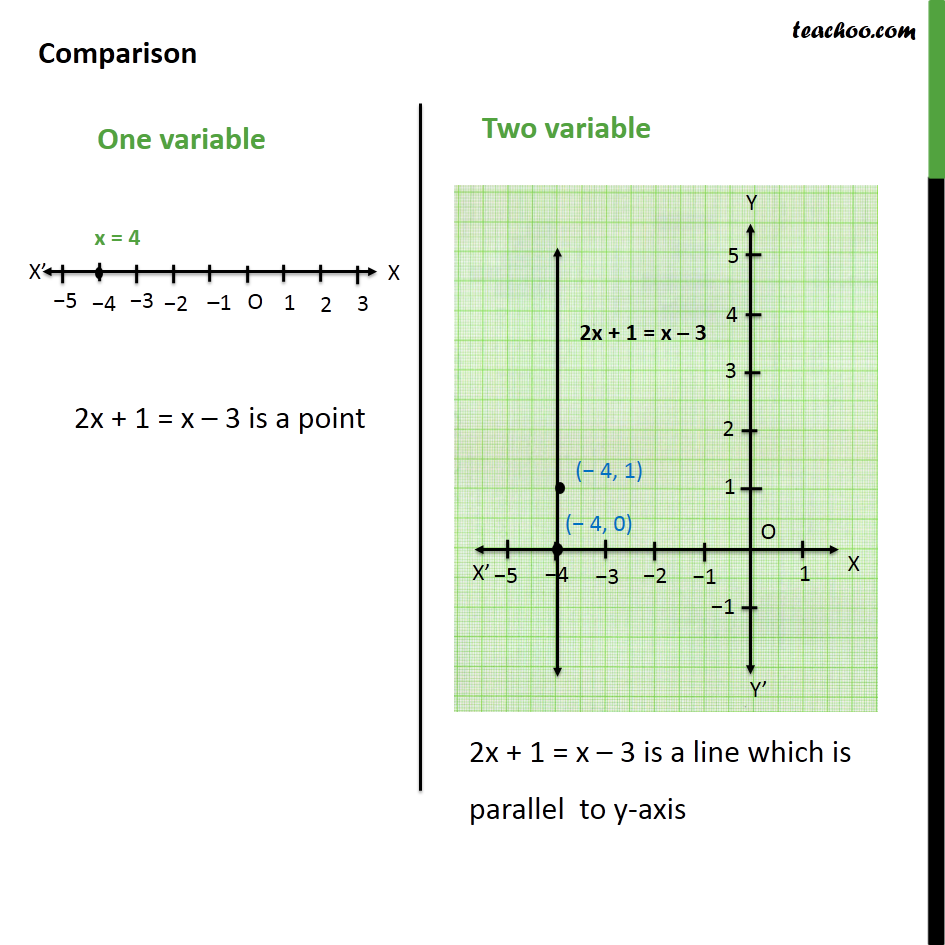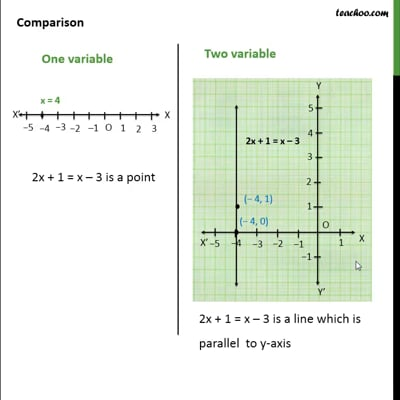This video is only available for Teachoo black users

Introducing your new favourite teacher - Teachoo Black, at only ₹83 per month

### Transcript

Example 9 Solve the equation 2x + 1 = x – 3, and represent the solution(s) on (i) the number line Number line is one variable In one variable, there is only x axis.(No y-axis) Solving 2x + 1 = x – 3 2x – x = –3 – 1 x = – 4 Here, 2x + 1 = x – 3 is a point Hence, we can say that in one variable 2x + 1 = x – 3 is a point Example 9 Solve the equation 2x + 1 = x – 3, and represent the solution(s) on (ii) the Cartesian plane. In Cartesian plane , we have x and y axis Hence, writing equation 2x + 1 = x – 3 in terms of x & y 2x + 1 = x – 3 2x – x = –3 – 1 x = –4 x + 0 = – 4 x + 0y = –4 Plotting points on graph Comparison